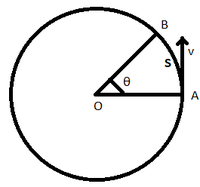GeeksforGeeks App
Open AppBrowser
Continue

Speed is simply as you know the measure of how fast or slow an object is moving, like how fast are you driving a car. Now, here we are talking of a specific type of speed. Angular speed is a type of speed only but here the body must move in a circular path.

### Angular Speed Formula

Angular speed is defined as the rate of change of angular displacement, that is the angle traversed by a body along a circular path. Angular speed is calculated in terms of a number of rotations/revolutions made by a body to the time taken. Angular speed is denoted by Greek letter, ‘ω’ known as Omega. The SI unit of angular speed is rad/s.

The angular speed is calculated using two different formula,

• ω = θ/t
• ω = v/r

### Formula Derivation

Let’s consider a body moving in a circular path with radius r shown above with a linear speed v. Let’s suppose that the body moves from point A to B covering a distance s through the circular arc and traversing an angle θ in time period t.Circular path covered by a body

As known the angular speed is rate of change of displacement – Angular speed, ω = θ/t

So the formula for angular speed is ω = θ/t .

Another formula for angular speed

Despite the formula stated above, there is another and more widely used formula for calculation of angular speed from the point of view of competitive exams.

As ω = θ/t ⇢ (1)

Now we know that distance moved across arc of a circle is equal to radius times angle traversed. So,

s = rθ

=> θ = s/r ⇢ (2)

From (1) and (2),

ω = s/(rt) ⇢ (3)

Also from general understanding of linear speeds,

v = s/t ⇢ (4)

From (3) and (4),

ω = v/r

### Sample Problems

Question 1: Consider a body moving along a circular path of radius 5m. It covers half revolution in 5s. Calculate its angular speed.

Solution:

In half revolution, the angle traversed is 180 degrees. In radians, it is equal to π radians.

ω = θ/t

=> ω = π/5 = 0.628 rad/s

Question 2: A car wheel of radius 2m is rotating with a linear speed of 10m/s. Calculate it’s angular speed.

Solution:

ω = v/r

ω = 10/2

Question 3: Consider a racing car travelling in a circular track with speed 18 km/hr and the radius of track is 0.2 m. Calculate the angular speed of the car.

Solution:

v = 18 km/hr = 5 m/s

r = 0.2 m

ω = v/r

= 5/0.2

Question 4: A car is moving along a circular path of radius 2m with an angular speed of 2 rad/s. Calculate the angle in degrees through which the car move in 2s.

Solution:

Given,  ω = 2 rad/s and t = 2s

Since ω = θ/t => θ = ωt

=> θ = (2 × 2) = 4 rad

In degrees, θ = 4 × (180/π) = 229.18 degree

Question 5: How many revolutions did a body make moving along a circular path with angular speed of 7π rad/s in 0.5s?

Solution:

Given ω = 7π rad/s and t = 0.5s

Since ω = θ/t => θ = ωt

θ = (7π × 0.5) = 3.5π

In 2π rad, revolutions covered is 1

=> In 1 rad, revolution covered is (1/2π)

=> In 3.5π rad, revolutions = 3.5π/2π = 1.75 revolutions

So, the body will complete 1 complete revolution and 3/4th of next revolution in time period of 0.5 s.

Question 6: What will be the angular speed of a body moving in a circular path of radius 2m which covers 4m of arc length 5s.

Solution:

Given s = 4m, r = 2m, t = 5s

Using formula s = rθ => θ = s/r

θ = 4/2 = 2 rad

Since ω = θ/t

=> ω = 2/5 = 0.4 rad/s

My Personal Notes arrow_drop_up
Related Tutorials